## Thursday, January 31, 2013

### Should CFO’s be Design Thinkers?

In recent Treasury Café posts we have focused on the topic of innovation:
In “Are You Sure You Want to Innovate?” we explored the realities of organizational forces and how an innovation effort often works at cross purposes to these, resulting in a situation that can be dangerous to your career.
In “Get Closer to Innovation With These 5 Questions” we took the role of a leader who desires innovation but is not directly involved on a day to day basis with a project or process.
Today we explore the underlying force that creates transformative innovations called “Design Thinking”. This is followed by some thoughts about how to deploy this within our organizations.

What is Design Thinking?
Design Thinking is both a process and a mindset.

Tim Brown and Jocelyn Wyatt describe the process side of Design Thinking in the Stanford Social Innovation Review as:
“The design thinking process is best thought of as a system of overlapping spaces rather than a sequence of orderly steps. There are three spaces to keep in mind: inspiration, ideation, and implementation. Think of inspiration as the problem or opportunity that motivates the search for solutions; ideation as the process of generating, developing, and testing ideas; and implementation as the path that leads from the project stage into people’s lives.”
Amir Khella gets to the mindset by answering the question “What Makes a Good Design Thinker?”:
·         “An observing eye and a constant sense of wonder
·         An empathetic attitude toward people’s behavior and habits
·         A questioning mindset that goes beyond the obvious
·         Patience to remain in problem space until the right questions are identified
·         A holistic approach to problem solving
·         The willingness to experiment and build
·         A passion for collaboration”

How Do We Implement Design Thinking?
There are literally hundreds of models that delineate the stages and factors that make up the design or innovation process. For those who want to explore some of these, the “How Do You Design?” manuscript from Dubberly Design Office will give you a great overview.
The Institute of Design at Stanford describes 5 modes that make-up the design process. These modes are iterative - we can bounce back and forth between them and among them in any order. In other words, it’s not linear.
These modes are:
·         Empathize
·         Define
·         Ideate
·         Prototype
·         Test
As we go through these five modes, they suggest that our activities follow the following guidelines:
·         Show Don’t Tell - Communicate your vision in an impactful and meaningful way by creating experiences, using illustrative visuals, and telling good stories.
·         Focus on Human Values - Empathy for the people you are designing for and feedback from these users is fundamental to good design.
·         Craft Clarity - Produce a coherent vision out of messy problems. Frame it in a way to inspire others and to fuel ideation.
·         Embrace Experimentation - Prototyping is not simply a way to validate your idea; it is an integral part of your innovation process. We build to think and learn.
·         Be Mindful Of Process - Know where you are in the design process, what methods to use in that stage, and what your goals are.
·         Bias Toward Action - Design thinking is a misnomer; it is more about doing than thinking. Bias toward doing and making over thinking and meeting.
·         Radical Collaboration - Bring together innovators with varied backgrounds and viewpoints. Enable breakthrough insights and solutions to emerge from the diversity.”
We will now explore each of the 5 modes in turn.

Empathize
The trait of empathy has manifested several times already throughout this post: in the mindset of Design Thinking, the modes of the Design Process, and in the activity guidelines.
Why is this?
At its core, Design Thinking is a human-centered process. And not just human centered as narrowly defined by the product or service we might be contemplating, but human in its holistic totality. Amir Khella notes “Design is not about products; it’s about people. Think beyond tasks; Their lives. Their challenges. Their dreams. The user’s journey starts long before they click that button.”
For this reason, it is natural that empathy is the place where we begin the design process - we need to understand the humanness of the user.
This is performed using several different techniques.
The first is to simply observe people – what they do, what they don’t do, and the context within which they do or don’t do it. Techniques from the field of ethnography are often deployed in order to accomplish this. Depending on the scope desired this can entail several months of intensive observation - Jan Chipchase and his team spent months and months in Afghanistan living with and observing people in relation to their use of mobile banking.
The second tactic we can perform is to engage with people, either through an interview process or what is termed ‘encounter’ processes. By asking the right set of questions we can gradually begin to understand the “whys” involved in the user’s process.
Finally, we can immerse ourselves in the customer experience, using the products and services, and experiencing their point of view. For example, someone designing a product for handicapped individuals might spend a week in a wheelchair in order to generate a reference experience.
The objective of the Empathize step is to develop a set of observations and experiences so as to develop understanding (or inspiration as the IDEO folks call it) of what user needs and desires require fulfillment.

Define
Once we have developed a wide range of experiences and understandings of our users, we undertake the Define step. Whereas in Empathize we are seeking as wide a range of information as possible, during Define we are in the process of consolidating and synthesizing the vast amount of data we have collected.
In Ron Moen’s perspective of the IDEO process, he names this step “Synthesis”, noting that:
“All information… is collected in the project room. This room becomes the key tool for translating the information into opportunities for design. Photographs, diagrams and drawings are all mounted on the wall to prompt discussion and illustrate key insights. The room becomes a tool for sorting and recording the ideas that develop.”
An essential thing to note about Define is that we are narrowing down a problem definition and scope through a synthesis process but not a solution. In fact, if we can “perfectly synthesize”, it would theoretically encompass the entire amount of information we had collected during Empathy!
A well-performed Define phase, according to the Institute of Design at Stanford, will generate “compelling needs and insights”, which will lead to ideas that allow us to “scope a specific and meaningful challenge”. This serves to inspire the team and provides a framework upon which to build as we perform other steps.

Ideate
Once we have one or more challenges as a result of the Define phase, we again embark on a step that is divergent, meaning that we will cast a wide net, move far afield in any number of directions, and entertain as many wild and crazy ideas that can be cooked up.
This phase is known as Ideate, and contrary to the old IBM commercial, it is not a silent personal experience but rather one that utilizes the “radical collaboration” noted earlier. The objective of this phase is both quantity and diversity in ideas.
There are a number of tools that can be used to accomplish this. Brainstorming is one that IDEO relies on heavily (in some interpretations of their process this step is called Brainstorming rather than Ideate). Story boards, where an idea is sketched out as a number of scenes in the users journey, is another useful process to consider.
People who have done career or “life-mission” exercises are familiar with the “write your obituary” process, which is yet another means of generating ideas that might be relevant to the challenge at hand.
For those interested in exploring other possible tools used to kick start idea generation, the Service Design Tools website has close to 20 to choose from.

Prototype
The Prototype phase is one where we take our ideas and convert them into something tangible and real.
What is somewhat different about this phase in the Design Thinking framework compared to others is that it is a “down and dirty” exercise rather than a “Beta” version, so to speak. In “The Art of Innovation”, IDEO’s David Kelly discussed how he, a significant customer, and others on the project team carried around a shaped block of wood as a prototype of the Personal Digital Assistant device. When encountering a situation where the device might be used, the person would literally take the block of wood out of their pocket and pretend to use it!
Because these are low-cost and low-tech, they allow rapid deployment and therefore rapid feedback, which lets one know whether they are on the right track or not. As IDEO’s Tim Brown notes in his book “Change by Design”, one of their maxims is “Fail early to succeed sooner”.
In addition to finding out what fails sooner rather than later, other benefits of the prototyping process involve learning, conversation and idea ignition, and as a means to discuss disagreements, perspectives, and possibilities that the prototype represents. Feedback from this process can provide additional insights to the other process phases – additional empathy for the user or new ideas for example.

Test
Whereas in the “normal” world testing is the step that precedes final deployment, in Design Thinking the Test phase, like all the phases, is one that is iterative with and between them. The maxim, as stated by the Institute of Design at Stanford, is “Prototype as if you know you’re right, but Test as if you know you’re wrong.”
Learning and refinement – of the user, the potential solutions, and/or our definitions - are some of the objectives of this step.
Ultimately, however, this is the phase that leads to deployment. In some versions of the Design Thinking process the final step is labeled Implementation.
One advantage to using the term Test instead is to illustrate that the design process is not over or final until the team is satisfied that it has developed something worthy of moving from the realm of concept and prototype to something that is in fact deployed.

Applying Design Thinking to the CFO’s Organization
Now that we have performed a brief survey of Design Thinking, we now turn to the CFO’s organization in order to discuss its usefulness.
The CFO organization involves relationships with people. A useful diagram of these is provided by Samuel Dergel, a leading executive recruiter. Given that relationships are fundamentally about people, an approach focused on human-centered design has a certain logical appeal.
Some possibilities of how Design Thinking might be deployed are:
Board of Directors
An organization’s Board of Directors gets a “package” prior to their meetings from Finance, which contains agendas, presentations, and other information as background to the upcoming discussions. What might come about if we used the Empathy step with this group?
Through formal interviewing, we might learn of their concerns with the company, their understanding of the dynamics and the financials, and their lingering but unanswered questions that they bring to the meeting because it was unanswered in the materials.
Through the observation technique, we might notice that although they receive the entire package at one time, they pace themselves and review it in increments over several days or weeks. We might notice that all the Board members make notes on notepads while they are reviewing the materials and they pack all materials when traveling to the meeting.
From these observations we might decide to send the “package” in increments, which alleviates extra effort on the part of staff to meet a singled deadline. We might redesign the materials so that there is room within them for note taking, simplifying their task by eliminating excess items.
Investors and Analysts
The investment community is another important constituent of the CFO organization.
As we have discussed in prior posts such as “Your Step by Step Guide to Calculating ROIC”, GAAP financials often do not present items in a way that allows us to view them from the cash perspective or on an “apples to apples” basis with other companies. In that post we ended up bouncing back and forth through a 10-K like a basketball!
Perhaps we can prototype and test a financial information delivery system that takes the relevant data for a set of ratios and provides that in one shot uploadable format. Under the collaboration sphere, perhaps we engage with these folks as we make decisions about which accounting policies to implement or which assumptions are selected.
A hint of these types of possibilities is Boeing’s recent announcement that they were going to make some alternative financial disclosures which disregarded the impact of pensions, since these can distort financial statements a great deal.
Business Unit and Function Partners
Treasury Strategies, in their most recent State of the Treasury Profession webinar, discussed the fact that the finance group is more and more a “critical partner” within the organization.
In partnering roles, the finance team is providing both information and services. Design Thinking can be used in this context to enhance the “Customer Journey” through “Engineered Experiences”. Tools within the Design Thinking toolkit, such as the Service Design Blueprint, can help us better assess and deliver relevant items to our business unit partners in ways that they will find valuable.
Imagine if everyone in the company looked forward to the budgeting process! We can make it so, if only we employ a bit of Design Thinking.

Key Takeaways
Design Thinking provides a mindset and toolkit for creating and implementing transformative innovation and improvements within organizations. Incorporating this within our finance organizations will allow us to create great change.
Questions
·         What issues do you currently face that a Design Thinking approach might be able to solve?
·         Have you had any experiences where the Design Thinking process was used?

Add to the discussion with your thoughts, comments, questions and feedback! Please share Treasury Café with others. Thank you!

## Wednesday, January 16, 2013

### Your Regression Results Step by Step

We introduced Regression Analysis last month in order to understand whether Apple’s earnings announcements were significant or not (see “Was Apple’s Earnings Announcement Really Important?”).
What we did not do was go in-depth into just what information regression analysis produces. Since this statistical technique is often used, it might be helpful to explore the output produced.
With this greater understanding we can make more informed decisions and ask better questions about the data we are looking at, and ultimately determine whether or not regression analysis is in fact the correct tool to use.

The Basic Output
Figure A is a screenshot of regression output from the R statistical program.
Figure A
Regression Output From R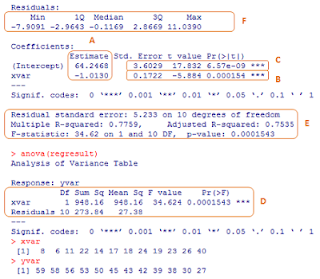This output has been divided into sections that we will reference throughout the post. The regression results in Figure A come from a data set in the book Statistical Methods, 8 ed. by Snedecor and Cochran (one of my favorites). The data is included in the graphic below the output (xvar and yvar).

The Estimates
The estimates of the regression equation are shown in the A box in Figure A. The regression equation (like any mathematical equation of a line shown in this form) is composed of two terms – the intercept and the slope. In Figure A the intercept is 64.2468 and the slope is -1.0130.
Figure B contains the set of equations that are involved in arriving at these values. Working from the bottom up, equations 6 and 7 are simply the averages of the X and Y data sets. The Y term,

Figure B
Regression Estimate Equations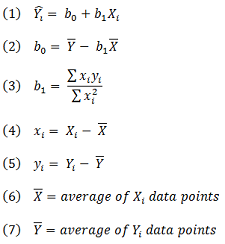which is on the other side of the “=” sign in equation 1, is the dependent variable and the X term is the independent variable. The bar symbol is used to depict averages, and the result is then often referred to as “X bar” or “Y bar”.

Once we have averages, we can calculate how much each individual data point varies from its average, which is shown in equations 4 and 5. For example, the 3rd X data point is 11, so in our equation notation we would say X3 = 11. The average of all X’s is 19, so X bar = 19, and thus the difference is 8, so x3 = 8 (note the upper case letters refer to the raw data and the lower case letters refer to the difference between the raw data and its average).
With these values we can calculate the slope estimate (b1) with equation 3, and the intercept estimate (b0) with equation 2.
The final regression equation is equation 1. The slope multiplies, or scales, the Xi data point, to which we then add the intercept, to come up with an estimate of the dependent variable for that data point. Using the 3rd one again, we come up with a Y value of 53.1039 (64.2468 - 1.0130 * 11). The symbol on top of the Y in equation 1 is called a “hat”, and thus it is the case that the estimated Y value is referred to as “Y hat”. Note that this is not the actual value of Yi (without the hat!). We’ll explore this soon.

Diving Into the Beta Estimate
In the B box of Figure A we are shown additional statistics for the b1 estimate, often referred to as the Beta estimate (for lovers of Greek letters!). The equations that calculate these values are

Figure C
Beta Estimate Equationsshown in Figure C.

Starting from near the bottom, equation 5, in the upper term, calculates the difference between the actual Y values and the fitted Y values (i.e. “Y hat” values). This difference is called the residual. The residuals are then squared and divided by the number of data points in the data set less 2. Finally, each individual calculation is added up to arrive at a total. This is the standard equation for variance.
Equation 4 takes the variance of the residuals that was just calculated and divides this by the sum of the variances of the x term only. This value is the variance of the b1 term, and equation 3 then takes the square root of this number. The result of equation 3 is shown in Figure A under the “Std. Error” column.
We can think about the math involved in this equation in order to understand the Beta estimate a little better. The standard error of the b1 term is 0.1722 (from Figure A). Now if the variance of x got smaller and smaller, so as to approach 0, then the standard error would become larger and larger (think 10/5 is 2, 10/4 is 2.5, etc.). If X hardly varies at all, while Y varies a great deal more, then the value of X as a predictor becomes more negligible. Because of this, we cannot have as much “confidence” that the b1 value provided is the “real” b1 value. For this reason, we must apply a larger margin of error for that term to grant more leeway for what the value might be.
Fortunately for us, Equation 2 helps us get to the same place. The t-value numerator is the difference between the estimate of the factor (b1) and the hypothesis that the factor does not matter (the “null” hypothesis:  β1 =0). The difference between these two numbers is then divided by the standard error of b1 (in effect scaling the difference). In our Figure A example the t-value of the b1 estimate is -5.884.
The t-value is then analyzed using a statistical distribution known as Student’s t. The inputs for this analysis are the t-statistic calculated above and the “degrees of freedom” used in the estimation process.
With the t-distribution we can do 2 things. First, we can calculate the probability that the t-value for the Beta estimate would occur even if the null hypothesis were in fact true (i.e. the “real” value of b1 was 0). This is known as the p-value  and is the value in last column of the B box of Figure A. Accordingly, we interpret this value as follows:
“The probability that we would calculate a beta estimate of -1.013 when the true value was 0 is 0.0154%”
This is a pretty low chance, so we are likely to conclude that the beta estimate from this regression equation matters.
The
Figure D
Beta Confidence Limits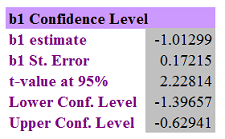This spreadsheet is available for download! And it contains all the equations covered in this post. Visit my Online Red Kettle for details. Hurry, it ends January 31st.
other thing we can do with the t-value is calculate the confidence limits of our b1 estimate. If we choose to say we want to be 95% confident of the estimate (a common threshold in statistics), we can convert this into the required t-value. We then take this t-value amount and multiply it by equation 3, and then both add and subtract this from our b1 estimate as shown in equation 1. Figure D shows the confidence levels for our equation calculated in Excel as -0.629 and -1.397. This means that even if b1 is not precisely -1.013 we can be pretty certain it lies somewhere in this range.

Diving Into the Alpha Estimate
In the C box of Figure A we are shown additional statistics for the b0 estimate, often referred to as the Alpha estimate (again, for lovers of Greek letters). The equations used for this are shown
Figure E
Alpha Estimate Equations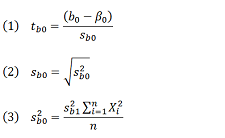in Figure E (note that some of the inputs are from the prior Beta set of equations).

Equation 3 shows that the variance of the b0 estimate is equal to the variance of the b1 estimate multiplied by the sum of all the independent variables squared, with the resulting calculation divided by the number of items in the data set. The square root of this value is then taken in equation 2, with the resulting figure in the first column of the C box in Figure A.
Equation 1 calculates the t-value for the alpha estimate using the null hypothesis (i.e.  β0 =0). With the t-value, in the same way
Figure F
Alpha Confidence Limitswe did in the prior section, we can a) calculate the probability that the value we arrived at would occur if the true value were 0, and b) the confidence limits of the Alpha estimate (shown in Excel format in Figure F).

The Analysis of Variance
In the D box of Figure A we are shown what is called an “Analysis of Variance Table”. This table divides up the regression results into those that can be attributed to the
Figure G
ANOVA Equations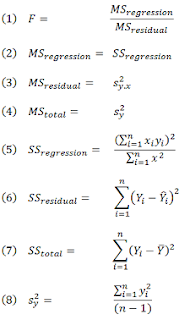Beta estimate and what remains for the residuals.

The equations involved in calculating this table are show in Figure G.
Equation 8 calculates the variance of the dependent variable data set by taking each result from equation 5 in Figure B, squaring it, and then adding all of these up. This total is then divided by the total number of data elements less 1.
Equation 7 calculates the sum total of the squared deviations in the Y data set (it is essentially the top half of Equation 8), while equation 6 calculates the sum total of the squared deviations of the Y residuals.  The only difference between these two calculations is whether or not Y has a “hat” or a “bar”.
The numerator of equation 6 multiples the difference in each X from its mean by the difference in each Y from its mean, and then squares the result. The denominator squares each X’s difference from its mean.
The “Sum Sq.” column is additive, meaning if we take the results of equation 6 and 7 and add them together we get equation 8, which provides a nice way to check our work.
Equations 2, 3 and 4 are used to populate the “Mean Sq.” column. Each one of these equations has been discussed previously in this post.  In addition, however, for the regression and residual items, each of these can also be calculated by taking the results in the “Sum Sq.” column and dividing by the number of degrees of freedom, again providing a check against our prior work up to this point.
Equation 1 calculates the F-statistic for the regression, which is the ratio of variance “explained” by our regression equation to the variance “left over” after this explanation. This statistic is then analyzed using the F-distribution. Similar to the t-distribution discussed in the Beta estimate section, we can then use this comparison to calculate the probability that the variance ratio of our regression would occur simply by chance assuming there was no statistical relationship.

Other Summary Results
The E box of Figure A shows a variety of data from the regression, and most of the elements in this box are items we have already seen.
The “Residual Standard Error” is simply the square root of equation 3 in Figure G. The F statistic data is the same as discussed in the last section.
The only new terms in this section are the r-squared and adjusted r-squared items. The equations
Figure H
Other Output Equations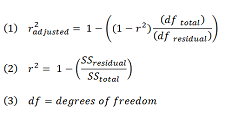for these are shown in Figure H. The inputs into these equations come from those in Figure G (equations 6 and 7) with the exception of the degrees of freedom.

If you follow the Wikipedia link you will see that degrees of freedom is a complex mathematical concept. For our purposes we will say that a degree of freedom is “used” to create a variable. For example, if we have 5 numbers and calculate an average, we use one degree of freedom, so there are 4 degrees of freedom remaining in the data set.
For a simple linear regression 2 degrees of freedom are used. For this reason, the degrees of freedom is the number of elements in
Figure I
Density Curve Comparisonthe data set minus 2.

Diving Into the Residuals
The residuals from a regression equation should be distributed normally with a mean of 0 and a standard deviation given by the standard error (the square root of Figure G’s equation 8).
The F box of Figure A shows a summary of the quartile distribution of the residuals. Since the mean of the residuals should be 0, the median of this value should also be close to 0. In our case we observe that the median data point is -0.1169, which
Figure J
Residual Plot: From Regressionis not too terribly different from 0 given that we only have 12 residuals to calculate the data from.

Figure I compares the probability density curve of the actual data to that of a random sample of data taken from a normal distribution with a mean of 0 and a standard deviation equal to that of the regression. Visually examining these lines shows us that the fit is fairly good.
A common means of visually testing normality is by observing the plot of residuals, which is shown in Figure J. Under a normal set of conditions, each quadrant in the graph should look similar to all the others from two perspectives – the number of data points and the number of extreme values.
In our
Figure K
Residual Plot: Normal Examplecase, the upper left and lower right quadrants contain more data points than the other 2, which might indicate a non-normal distribution to the residuals. In addition, the more extreme value points are also contained in those quadrants.

For comparison, Figure K is a graph of 1,000 randomly generated data points with a mean of 0 and a standard deviation of 1. As you can see, upon visual inspection no quadrant appears to be unduly heavy or light with data points. In addition, extreme values exist in all the quadrants as well.
Finally, a QQ plot compares the residuals to known theoretical distribution. Figure L plots the residuals from our regression in orange, and compares that to a normal distribution with a mean of 0 and a standard deviation equal to 5.233 (the standard error as discussed above and shown in the E box of Figure A). The residuals should lie close to the line in order to be a good fit.
For
Figure L
QQ Plotsome examples of what QQ plots look like when things are not normal go to the Murdoch University website (where they make the claim “A sufficiently trained statistician can read the vagaries of a Q-Q plot like a shaman can read a chicken's entrails”!).

There are equation forms of testing as well, called “Goodness of Fit” tests, which we will save for later.

In many cases we are in the role of consuming regression data rather than creating it. Some examples might be when we are: a) members of a project selection or steering committee where several groups are attempting to “pitch” their solutions, or b) meeting with bankers or consultants with products/services to sell, or c) supervising individuals/groups who are performing the actual analytical work.
In these situations we are in a position where we do not know as much as those we are communicating with, which can make it difficult to ascertain whether the decisions we might be led to make are based on good information or not.
Some questions that will help us “get comfort” with the analysis are:

1. What Evaluations Did You Perform With the Residuals and What Were the Results?
For linear regression, residuals need to be normal in order for us to use t-tests of significance, confidence levels, r-squares and probability estimates among other things. These estimators and tests are incorrect if the residuals are not normal.
Non-normal distributions might be due to too little data. Other times it might mean there is an underlying data structure that requires different tools to assess. We might be omitting significant independent variables that play a critical
Figure M
Linear vs. Quadratic Comparisonrole in determining what the Y values should be.

Figure M shows regression results for 2 data sets, both driven by the same variable. As you may notice, while the QQ plots may look fairly good, the residual plots clearly show that the right hand data set has some curvature to it. If we used the left hand equation to make future estimates, we would under-predict the extremes and over-predict the middles on a consistent basis.
A puzzled look when asked this question, or a mention of some other factor like “the r-squares were so high”, or hemming and hawing all indicate that testing the normality assumption was not sufficiently performed.
Folks who have tested for normality should be able to produce or discuss the various plots talked about above and what they indicated, and other goodness of fit testing that was conducted as well.

2. How Were Outliers Handled and Why?
Another reason to examine residuals is to identify potential problems with our data. Sometimes one or two points will “stick out like a sore thumb”. These require further investigation.
Perhaps there was a data entry error such as a transposition of two numbers or the omission of a decimal point.  Perhaps there was a one-time unusual event. If we are regressing earnings numbers sometimes we pick up those special charges that we did not intend to.
However, in pursuit of getting “better” regression results, we may sometimes label something an “outlier” and on that basis omit it from the data set used to create
Figure N
Outlier Impacts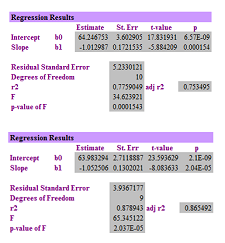This spreadsheet is available for download! And it contains all the equations covered in this post. Visit my Online Red Kettle for details. Hurry, it ends January 31st.
the regression results. This is dangerous if the data truly should be concluded.

Figure N shows the “before and after” once the 3rd set of data elements from the Figure A regression is eliminated. All the statistics improve – t-values are higher, the residual error is less, the r-squared values are higher. Yet, without a compelling reason to do so, simply eliminating data to make the regression stats better is not a very good analysis.
Asking this question should surface if any outliers were eliminated and the reasoning behind them, allowing you to also participate in evaluating whether an outlier truly is just such a thing. One must do this with caution – just because something is a rare occurrence, such as a stock market bubble or crash, doesn’t mean they are an outlier. As history has shown, bubbles and crashes are in fact repeating events.

3. Why Are the Variables Selected Appropriate?
Because we use terms such as independent and dependent variable, and we make statements about the results such as the “change in this value is associated with a change in that value”, and there is an underlying human tendency to create cause and effect explanations even when there are not any, we can be vulnerable to associating meaning to the analysis when we should not.
There is an old saying in analysis that “correlation is not causation”. For example, let’s consider having a cough and a stuffy nose. If we regress one of these factors on another we will likely get very good regression statistics. Yet, did our cough cause our stuffy nose? Did our stuffy nose cause our cough? In all likelihood the
Figure O
What Causes What?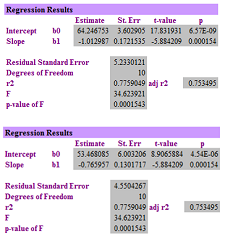This spreadsheet is available for download! And it contains all the equations covered in this post. Visit my Online Red Kettle for details. Hurry, it ends January 31st.
cause is a variable not part of our analysis – a cold or flu virus!

Figure O shows the results of our original Figure A regression compared to the results when the data is “flipped” – in other words what used to be X is now Y, and what is Y is now X. The values for the estimates are slightly different, but the r-squared and F statistics are exactly the same and indicate high probability.
So, does X cause Y, or does Y cause X? Both are plausible solutions. Determining the circumstances under which we can determine causation is a qualitative judgment, and likely one that should be reviewed.

4. Are Variables Measuring What We Want Them To?
Given that access to data is sometimes difficult, there is a temptation to use the data that is provided. However, depending on what we hope to learn from our analysis it might not be in the best format…or it might not be appropriate at all!
There are times when we want to understand the changes in things. For example, we need to construct a hedge ratio for a particular risk we wish to mitigate. In this case it is the changes that we need to protect against. If corn is \$2 or if it is \$6, if it moves by 30 cents I want my hedge instrument to move in likewise fashion.
So to examine how this can impact things, I took the original data set and converted it to percentage changes. This means that if the original set had X at 25 and Y at 40, and the next X value was at 30 and the next Y value at 42, then the transformed
Figure P
Datatype ImpactThis spreadsheet is available for download! And it contains all the equations covered in this post. Visit my Online Red Kettle for details. Hurry, it ends January 31st.
data set would be X = 20% ((30-25)/25) and Y= 5% ((42-40)/40).

Figure P shows how this change in data dramatically impacts our results. From a relatively high r-square in the 70’s we go to one close to 0, essentially no relationship between the movement of X and the movement of Y!
And all due to simply transforming the existing data.

Key Takeaways
Regression analysis is comprised of a series of equation and assumptions. It can be used to generate extremely useful and valuable insights, but those who are going to rely on this output to support important decisions need to dig into the results in order to determine whether the analysis is in fact reliable or not.
Questions
·         What questions would you recommend someone ask when presented with the output of a regression analysis?

Add to the discussion with your thoughts, comments, questions and feedback! Please share Treasury Café with others. Thank you!